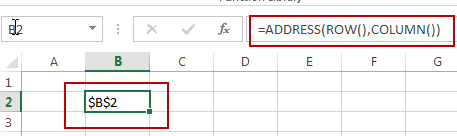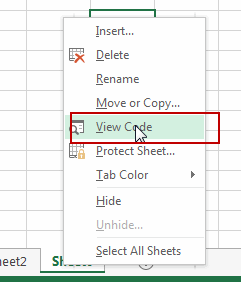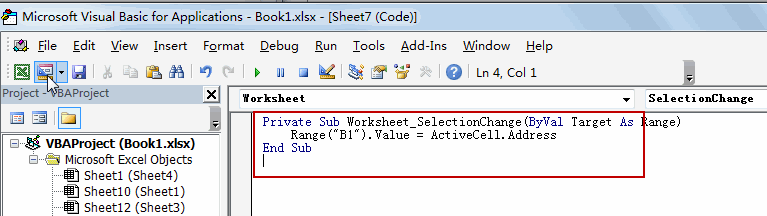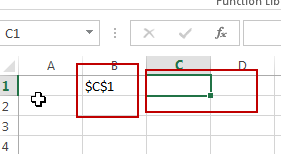# How to Get the Active Cell Address in Excel

This post will guide you how to get the active Cell address with a formula in Excel. How do I return address of active cell with a VBA Macro in Excel.

## Get the Active Cell Address with Formula

If you want to return the address of an active cell in your current worksheet, you can use a formula based on the ADDRESS function, the ROW function and the COLUMN Function to achieve the result. The formula is shown as below:

`=ADDRESS(ROW(),COLUMN())`

You just need to select a cell and make it as active cell, then enter this formula into the formula bar, press Enter key on your keyboard. You would notice that the address of active cell is returned.## Get the Active Cell Address with VBA

You can also use an Excel VBA Macro to achieve the same result of returning the active cell address to a given cell. You just need to do the following steps:

#1 right click on the sheet tab in your worksheet, and select View Code from the popup menu list. And the Microsoft Visual Basic for Application window will appear. Or you can directly press Alt+ F11 to launch the VBA window.#2 type the following code into the code window, then click Save button to save it.

```Private Sub Worksheet_SelectionChange(ByVal Target As Range)
End Sub```#3 close VBA window and back to your worksheet. You can try to click or select one cell, the cell address of active cell is shown in Cell B2.### Related Functions

The Excel ADDRESS function returns a reference as a text string to a single cell.The syntax of the ADDRESS function is as below:=ADDRESS (row_num, column_num, [abs_num], [a1], [sheet_text])….
• Excel COLUMN function
The Excel COLUMN function returns the first column number of the given cell reference.The syntax of the COLUMN function is as below:=COLUMN ([reference])….
• Excel ROW function
The Excel ROW function returns the row number of a cell reference.The ROW function is a build-in function in Microsoft Excel and it is categorized as a Lookup and Reference Function.The syntax of the ROW function is as below:= ROW ([reference])….

Related Posts
VBA Macro For VLOOKUP From Another Sheet

In the previous post, you should know that how to fix or remove the #N/A error when using VLOOKUP formula to lookup value from another sheet. And this post will show you how to use VBA code to vlookup data ...

How To Insert Comments in Protected Worksheet in Excel

This post will show you how to allow comments in a protected worksheet in Excel. You can easily to insert comments into cells in a normal worksheet in Excel, but if want to insert a comment in a worksheet that ...

How To Convert Text to Upper Cases(Using VBA) in Excel

This post will show you how to switch from lower case to upper case in Excel. and I am going to show you two different ways of converting text to upper cases using formula or VBA macro in Excel 2013,Excel ...

How To Hide Every Other Row in Excel (Using VBA)

This post will show you how to hide alternate rows or columns in Excel or how to hide every third, fourth, fifth row or column in Excel. If you want to hide every other row in your current worksheet, how ...

How to Disable the Save As Prompt in Excel

This post will show you how to use a VBA Macro to save an Excel file and overwrite any existing file without a prompt so that you are going to get the little window that says file already exists do ...

How to Count Cells that Contain even or odd numbers in Excel

This post will guide you how to count the number of cells that contain odd or even numbers within a range of cells using a formula in Excel 2013/2016.How do I count cells that contain odd numbers through the use ...

How to Count Cells that Contain negative Numbers in Excel

This post will guide you how to count the number of cells that contain negative numbers within a range of cells using a formula in Excel 2013/2016.You can count the number of negative numbers in your data using easy functions ...

How to Count Cells Are Not Blank or Empty in Excel

This post will guide you how to count cells that are not blank or empty in a given range cells using a formula in Excel 2013/2016.How do I count the number of cells that are not blank in a particular ...

How to Count Cells Not equal to X or Y in Excel

This post will guide you how to count the number of cells not equal to criteria X or Y in a given range cells using a formula in Excel 2013/2016.You can easily to count cells equal to or not equal ...

How to Count Cells Less Than a Specific Value in Excel

This post will guide you how to count the number of cells less than a particular numeric value in a given range cells using a formula in Excel 2013/2016. How do I count cells that are less than a specific ...

Sidebar